Function Repository Resource:

# EulerizeGraph

Add edges to a graph to make it Eulerian

Contributed by: Peter Burbery
 ResourceFunction["EulerizeGraph"][graph] adds edges to a connected graph to make it Eulerian.

## Details

An Eulerian graph is also referred to as unicursal.
A graph is Eulerian if it contains a path through all edges with no repetitions.
If the input graph is already Eulerian, it is returned unchanged.

## Examples

### Basic Examples (2)

Create the bridges of Königsberg graph:

 In:=Out=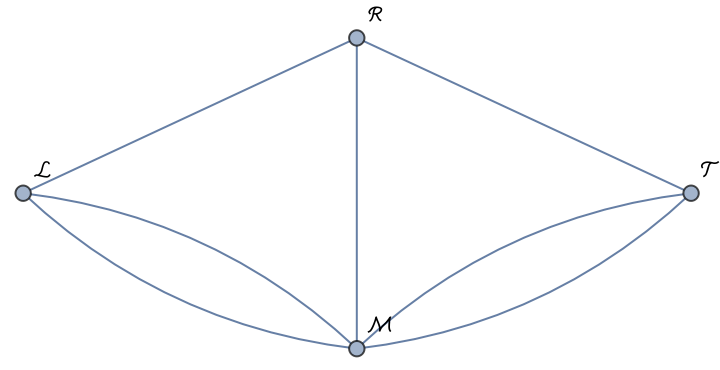Eulerize the Königsberg graph:

 In:=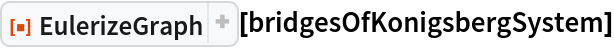Out=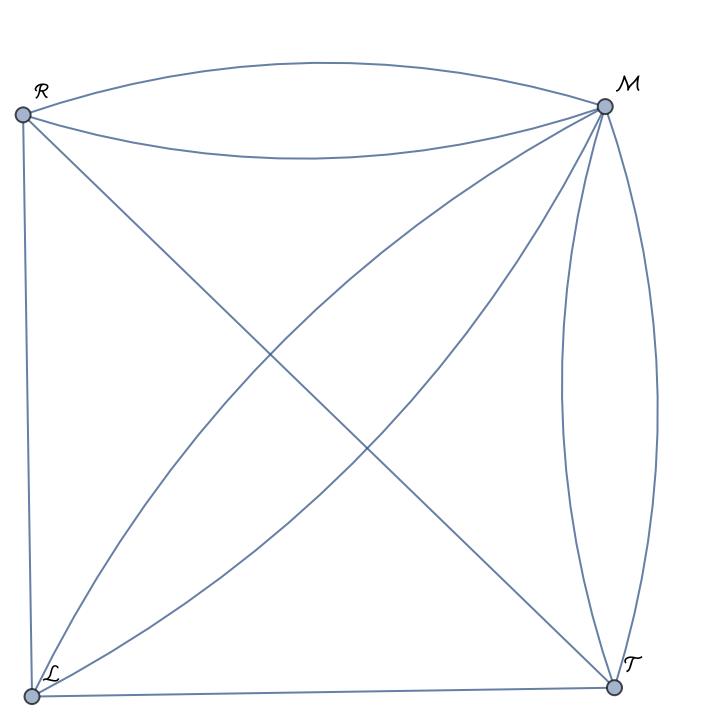Eulerize the graph corresponding to the modern-day bridges of Königsberg (some of the original bridges are no longer present):

 In:=Out=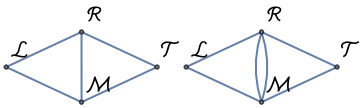Show the Pappus graph and its Eulerized counterpart:

 In:=Out=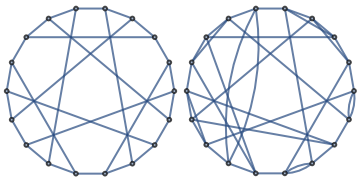### Properties and Relations (3)

If a graph is already Eulerian, the graph remains unchanged:

 In:=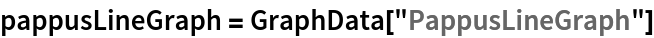Out=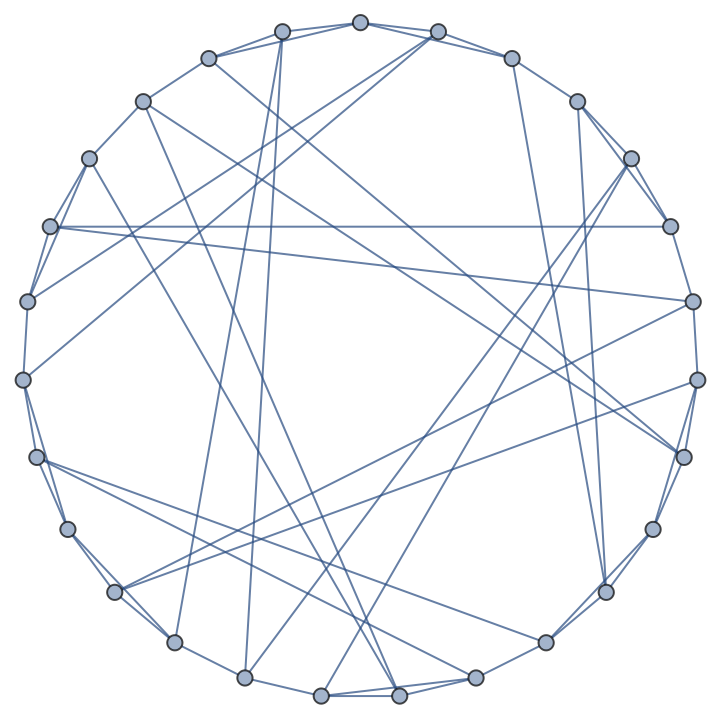Check that it is Eulerian:

 In:=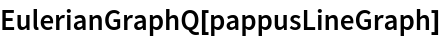Out=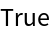Check that the original and Eulerized graphs are identical:

 In:=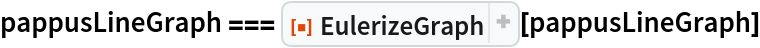Out=Peter Burbery

## Version History

• 1.0.0 – 04 May 2022